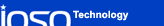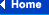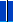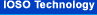##### Available options of using IOSO tools
• Currently the user has the ability to use the complete set of IOSO tools for solving the following practical problems:
• Optimization problem solving for finding the most efficient technical solutions, when you supply us the executable analysis code for your system;
• Joint software development that will include our optimization module as a part;
• Making use of our consulting services to formulate your particular optimization problem, to develop the most efficient problem solution strategy that minimizes your time and cost;
• Real-life object experimental optimization (without mathematical model usage);
• Development of the specialized software for optimizing the process of conducting physical experiments.
##### General requirements and characteristics of IOSO Technology algorithms:
 Problem dimensionality Moderate dimension implies up to 20-50 design variables. Large dimension implies up to 100-200 design variables. Nonlinear inequality constraints For problems of moderate dimension the number of inequality constraints should be up to 20-50. For large dimension problems, there is no limit on the number of inequality constraints. Nonlinear equality constraints Each equality constraint should be transformed into two inequality constraints. Alternatively, the penalty function approach could be used. Any types of penalty functions could be employed, including discontinuous ones. The reason for such a variety is that our optimization algorithms are insensitive to the topology of the objective function. The number of objective functions For problems of moderate dimension the number of objectives should be up to 10. For large dimension problems up to 20-40. If the user will require, this number could be increased. The number of processors that can be used with parallel optimization algorithms No restrictions. For example, for 5 design variables problem 2, 9, 23, 100, and more processors can be employed. The number of levels that can be used in multilevel optimization algorithms Up to 3 levels of mathematical models can be used: low fidelity models (LFM), moderate fidelity models (MFM), and high fidelity models (HFM). Their use is adaptively determined by our response surface algorithms without users input. MFM and HFM mathematical models should be developed by the user. For example, MFM 2D CFD, HFM 3D CFD. If the user requires, more fidelity levels can be employed. For example, 2D CFD, quasi 3D CFD, and 3D CFD. Also, the difference in the fidelity of the mathematical model could be determined by the discretization level only. Requirements for the topology of the objective function and constraints There are no restrictions on topology of the objective function and constraints except for one: the capability to determine the value of the objective function and constraints at least in some subspace of the design space. Requirements for a starting point of optimization process No starting point is required. Use of previously obtained information in optimization process. It is possible in the optimization process to make use of the information obtained prior to the optimization, including use of databases. Specifics of the optimization process dynamics It is possible to stop the optimization process at any iteration and then ontinue it at any other time without any extra information from the user. It is also possible to change the method of optimization when starting the continuation process. In the last case, however, the user may be required to provide some extra information. Information about existence of the solution in the design space There is no need to provide any information about existence of the solution in the design space. In case of incorrectly posed optimization problem (no feasible solution), the algorithms will find the solution with minimal possible violation of the constraints. Requirements to the computational reliability of the analysis program Our optimization process is very insensitive to fluctuations in computational process. That is why it is allowed for the users analysis program to fail during optimization process. It is also allowed to have some regions in the design space where it is not possible to evaluate objective function and constraints. In this case the only requirement for the analysis program is not to go into the infinite loop in these regions. Requirements to the user The user has to be able to correctly formulate an optimization problem. Particularly, Choose the objective functions (optimization criteria); Define constraints; Choose design variables and their allowable bounds; Prepare the analysis code for information exchange with optimization module: be able to get the design variables from the optimization module and provide objective function and constraints to the optimization module. It is not required to specifically modify analysis code when preparing for integration with optimization module. That is why integration goes fast. Requirements to knowledge of optimization procedure IOSO Technology control parameters are defined within the algorithm and are adaptively changed during optimization process without users interference. Requirements to programming language of the analysis code The information exchange between users analysis code and optimization module is accomplished by means of external files. That is why analysis code can be written in any programming language.
##### Optimization rezults
• Extremal value of the objective function for single-objective formulation or Pareto set for multi-objective formulation.
• Vector of design variable values providing the best objective function for single-objective formulation. Set of vectors corresponding to Pareto set for multi-objective formulation.
• Constraint values at the optimal point.
• Set of points in the design space that are close to the optimal point.

The user can take into account this information when analyzing the optimal solution. Particularly, the user may choose some other point, rather than the optimal, as the best point, according to his other considerations. The objective function value at the chosen point, however, will have the value close to the one at the optimal point. This information may also help in formulating new optimization problems at a later stage, which results in cost reduction for obtaining the new solution.

##### Naming Convention for IOSO Technology Algorithms

IOSO Technology algorithm names follow the pattern IOSO [Robust] N/P/L/R S/M [Gt] x.x , where:

• Robust   - corresponds to algorithms for robust design optimization and robust optimal control.
• First letter is the algorithm type:
• P - parallel optimization;
• L - multilevel optimization with adaptive change of the object model fidelity (low-, middle-, high- fidelity models);
• R - multilevel parallel optimization.
• Second letter is the algorithm class:
• S - single-objective optimization;
• M - multi-objective optimization.
• Gt  - corresponds to algorithms for large dimensionality problems (hundreds of variables).
• x.x  - is the algorithm version.

For example,

 IOSO LS Gt 1.0 is the 1.0 version of the algorithm for solving single objective optimization problems of large dimension using multilevel strategy. IOSO Robust NM 5.1 is the 5.1 version of the algorithm for solving multiobjective robust design optimization problems of moderate dimension.

Back...